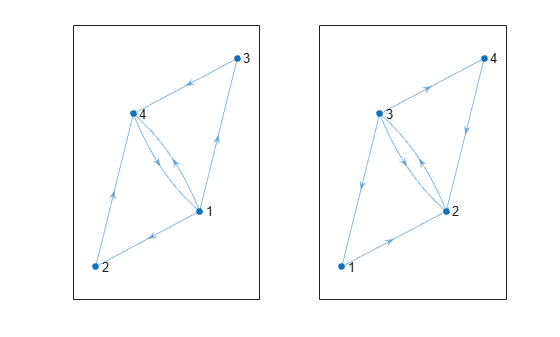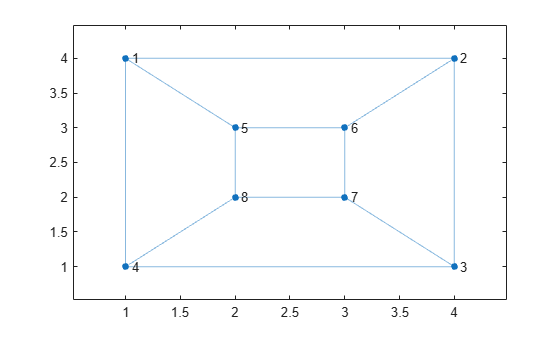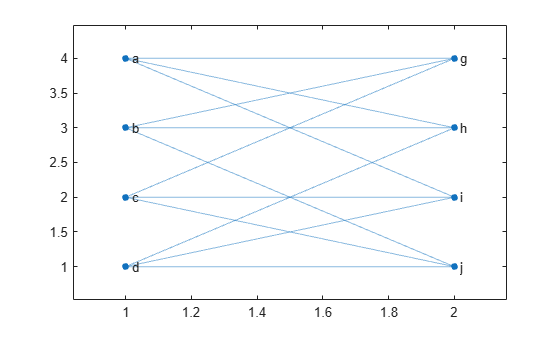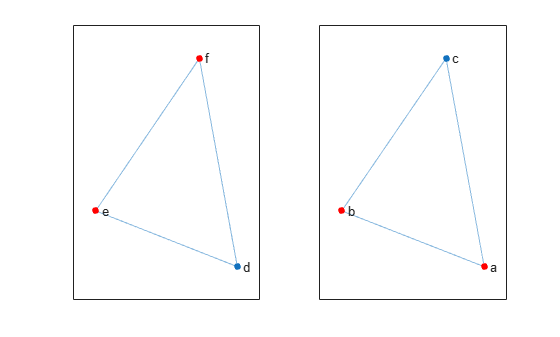# isomorphism

## 语法

``P = isomorphism(G1,G2)``
``P = isomorphism(___,Name,Value)``
``[P,edgeperm] = isomorphism(___)``

## 说明

``P = isomorphism(G1,G2)` 计算图 `G1` 和 `G2` 之间的图同构等价关系（如果存在）。如果不存在同构，则 `P` 为空数组。`

``P = isomorphism(___,Name,Value)` 使用一个或多个名称-值对组参数指定其他选项。例如，您可以指定 `'NodeVariables'` 和一个节点变量列表，以指示同构必须保留这些变量才有效。`
``[P,edgeperm] = isomorphism(___)` 还返回边置换 `edgeperm` 的向量。此输出使您能够在处理多重图时保留边变量。`

## 示例

```G1 = digraph([1 1 1 2 3 4],[2 3 4 4 4 1]); G2 = digraph([3 3 3 2 1 4],[1 4 2 3 2 2]); subplot(1,2,1) plot(G1) subplot(1,2,2) plot(G2)````p = isomorphism(G1,G2)`
```p = 4×1 3 1 4 2 ```

```G1 = graph([1 1 1 2 2 3 3 4 5 5 7 7],[2 4 5 3 6 4 7 8 6 8 6 8]); plot(G1,'XData',[1 4 4 1 2 3 3 2],'YData',[4 4 1 1 3 3 2 2])``````G2 = graph({'a' 'a' 'a' 'b' 'b' 'b' 'c' 'c' 'c' 'd' 'd' 'd'}, ... {'g' 'h' 'i' 'g' 'h' 'j' 'g' 'i' 'j' 'h' 'i' 'j'}); plot(G2,'XData',[1 2 2 2 1 2 1 1],'YData',[4 4 3 2 3 1 2 1])````p = isomorphism(G1,G2)`
```p = 8×1 1 2 5 3 4 7 6 8 ```

```G1 = graph({'d' 'e' 'f'},{'e' 'f' 'd'}); G1.Nodes.Color = {'blue' 'red' 'red'}'; G2 = graph({'a' 'b' 'c'},{'b' 'c' 'a'}); G2.Nodes.Color = {'red' 'red' 'blue'}';```

```subplot(1,2,1) p1 = plot(G1); highlight(p1,{'e' 'f'},'NodeColor','r') subplot(1,2,2) p2 = plot(G2); highlight(p2,{'a' 'b'},'NodeColor','r')````p = isomorphism(G1,G2)`
```p = 3×1 1 2 3 ```

`p = isomorphism(G1,G2,'NodeVariables','Color')`
```p = 3×1 3 1 2 ```

`[G1.Nodes.Name, G2.Nodes.Name(p)]`
```ans = 3x2 cell {'d'} {'c'} {'e'} {'a'} {'f'} {'b'} ```

## 输入参数

`G1``G2` 必须同为 `graph` 对象，或者同为 `digraph` 对象。

## 输出参数

```[p,edgeperm] = isomorphism(g1,g2,'EdgeVariables',edgevars); g2perm = reordernodes(g2, p); g2perm.Edges(:, 2:end) = g2perm.Edges(edgeperm, 2:end); ```